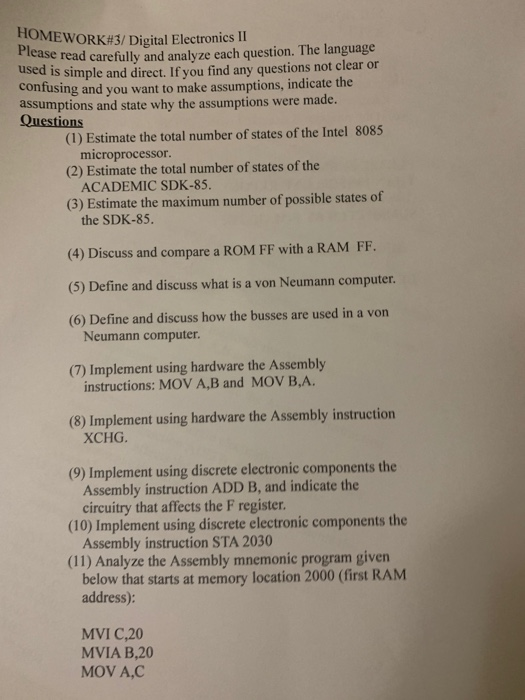# Question HOMEWORK#3/ Digital Electronics II Please read carefully and analyze each question. The language used is simple and direct. If you find any questions not clear or confusing and you want to make assumptions, indicate the assumptions and state why the assumptions were made. Questions (1) Estimate the total number of states of the Intel 8085 microprocessor (2) Estimate the total number of states of the ACADEMIC SDK-85. (3) Estimate the maximum number of possible states of the SDK-85. (4) Discuss and compare a ROM FF with a RAM FF. (5) Define and discuss what is a von Neumann computer. (6) Define and discuss how the busses are used in a von Neumann computer. (7) Implement using hardware the Assembly instructions: MOV A,B and MOV B.A. (8) Implement using hardware the Assembly instruction XCHG (9) Implement using discrete electronic components the Assembly instruction ADD B, and indicate the circuitry that affects the F register. (10) Implement using discrete electronic components the Assembly instruction STA 2030 (11) Analyze the Assembly mnemonic program given below that starts at memory location 2000 (first RAM address): MVI C,20 MVIA B,20 MOV AC85JWFB The Asker · Electrical EngineeringTranscribed Image Text: HOMEWORK#3/ Digital Electronics II Please read carefully and analyze each question. The language used is simple and direct. If you find any questions not clear or confusing and you want to make assumptions, indicate the assumptions and state why the assumptions were made. Questions (1) Estimate the total number of states of the Intel 8085 microprocessor (2) Estimate the total number of states of the ACADEMIC SDK-85. (3) Estimate the maximum number of possible states of the SDK-85. (4) Discuss and compare a ROM FF with a RAM FF. (5) Define and discuss what is a von Neumann computer. (6) Define and discuss how the busses are used in a von Neumann computer. (7) Implement using hardware the Assembly instructions: MOV A,B and MOV B.A. (8) Implement using hardware the Assembly instruction XCHG (9) Implement using discrete electronic components the Assembly instruction ADD B, and indicate the circuitry that affects the F register. (10) Implement using discrete electronic components the Assembly instruction STA 2030 (11) Analyze the Assembly mnemonic program given below that starts at memory location 2000 (first RAM address): MVI C,20 MVIA B,20 MOV AC
More
Transcribed Image Text: HOMEWORK#3/ Digital Electronics II Please read carefully and analyze each question. The language used is simple and direct. If you find any questions not clear or confusing and you want to make assumptions, indicate the assumptions and state why the assumptions were made. Questions (1) Estimate the total number of states of the Intel 8085 microprocessor (2) Estimate the total number of states of the ACADEMIC SDK-85. (3) Estimate the maximum number of possible states of the SDK-85. (4) Discuss and compare a ROM FF with a RAM FF. (5) Define and discuss what is a von Neumann computer. (6) Define and discuss how the busses are used in a von Neumann computer. (7) Implement using hardware the Assembly instructions: MOV A,B and MOV B.A. (8) Implement using hardware the Assembly instruction XCHG (9) Implement using discrete electronic components the Assembly instruction ADD B, and indicate the circuitry that affects the F register. (10) Implement using discrete electronic components the Assembly instruction STA 2030 (11) Analyze the Assembly mnemonic program given below that starts at memory location 2000 (first RAM address): MVI C,20 MVIA B,20 MOV AC